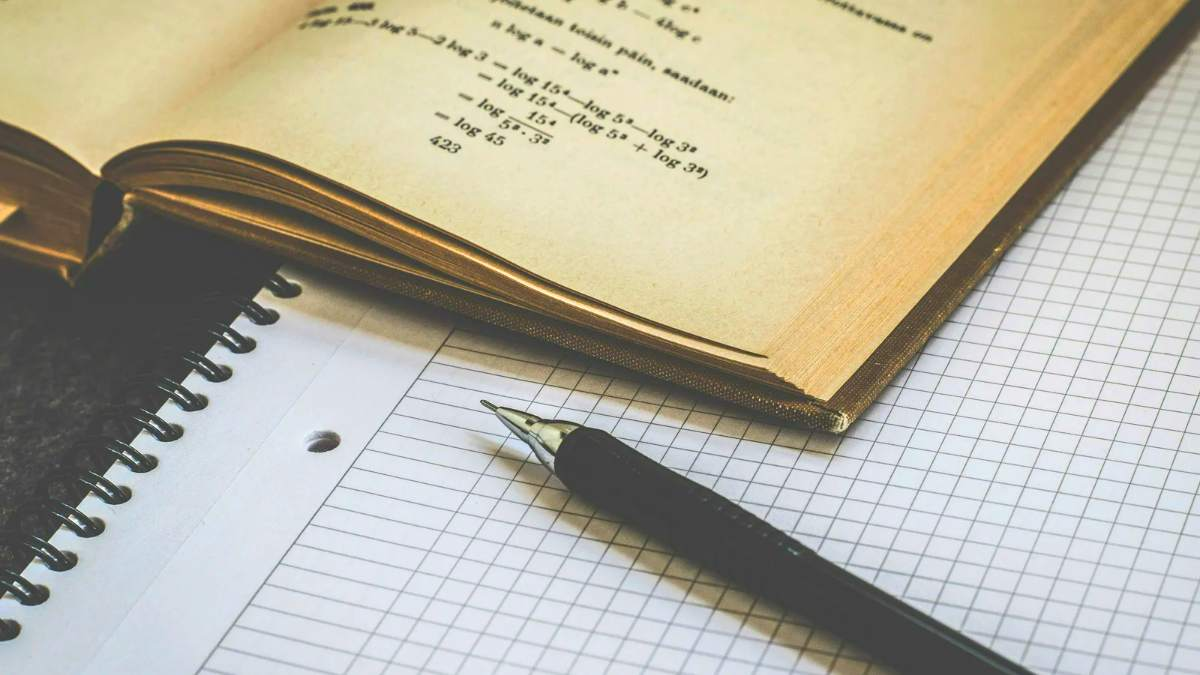# Math Riddle For Exams: 5 Minute-Problems On Time, Speed, And Distance.

Math riddles for exams: Today we have prepared 5 math questions on calculating time, speed, and distance so you can ace your tests and exams. Try and solve the questions here.Math Riddle For Exams: 5 Minute-Problems On Time, Speed, And Distance.

Solving math riddles on the internet might not be on your to-do list, but exams are nearing and math is easily one of the most difficult subjects to master. So, here we are a series of math riddles to help you prepare for any math exam, academic or competitive. This time we have 5 math problems from around the web on time, speed, and distance.

Let’s begin the test.

Wait! Go on your phone and set a timer to 60 seconds. Try to solve each question in under 60 seconds. So, overall you have 5 minutes to solve these 5 math riddles.

Let’s get started.

### Math Riddle For Exams: 5 Minute-Problems On Time, Speed, And Distance.

Math riddle #1

Walking at ⅚th of his usual speed, Mike reached his destination 10 minutes late. Find his usual time, and the time taken on this occasion.

Math Riddles With Answers: Can You Solve These Two Basic Math Problems?

Math riddle #2

A person travels from one place to another at 30 km/hr and returns at 120 km/hr. If the total time taken is 5 hours, then find the Distance traveled.

Math riddle #3

A car takes 4 hours to cover a distance if it travels at a speed of 40 mph. What should be its speed to cover the same distance in 1.5 hours?

Try And Solve This Basic Math Riddle That Has Stumped Even Engineers.

Math riddle #4

An airplane covers a certain distance at a speed of 240 km/h in 5 hours. To cover the same distance in 1 hour, it must travel at what speed?

Math riddle #5

After traveling 50km, a train meets with an accident and travels at ¾ of its usual Speed and reaches 45 minutes late to its destination. Had the accident happened 10km further on it would have reached 35 min late. Find the usual Speed of the train.

Math Riddle: Even Math Teachers Have Failed To Solve This Viral Stolen Bill Problem. Can You?

Math riddle #1

Mike’s usual time is 50 minutes and on the occasion, he took 50 + 10 = 60 minutes

Math riddle #2

The distance traveled is 120 km.

Math Riddle: Can You Solve This Children's Math Problem That Has Puzzled Even Adults?

Math riddle #3

To cover the distance in 1.5 hours, the car’s speed must be 106.66 m/h (miles per hour).

Math riddle #4

To cover the distance in one hour, the airplane must travel at the speed of 720 km/h.

Math riddle #5

The usual speed of the train is 20 km/h.

Math Riddle IQ Test: What Fraction Of The Shape Is Shaded? 99% Have Failed.

We hope you liked these math riddles on calculating time, speed, and distance.